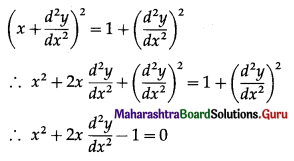Balbharti 12th Maharashtra State Board Maths Solutions Book Pdf Chapter 6 Differential Equations Ex 6.1 Questions and Answers.

## Maharashtra State Board 12th Maths Solutions Chapter 6 Differential Equations Ex 6.1

1. Determine the order and degree of each of the following differential equations:

Question (i).
$$\frac{d y}{d x^{2}}+X\left(\frac{d y}{d x}\right)+y=2 \sin x$$
Solution:
The given D.E. is $$\frac{d y}{d x^{2}}+X\left(\frac{d y}{d x}\right)+y=2 \sin x$$
This D.E. has highest order derivative $$\frac{d^{2} y}{d x^{2}}$$ with power 1.
∴ the given D.E. is of order 2 and degree 1.Question (ii).
$$\sqrt{1+\left(\frac{d y}{d x}\right)^{2}}=\frac{d^{2} y}{d x^{2}}$$
Solution:
The given D.E. is $$\sqrt{1+\left(\frac{d y}{d x}\right)^{2}}=\frac{d^{2} y}{d x^{2}}$$
On cubing both sides, we get
$$1+\left(\frac{d y}{d x}\right)^{2}=\left(\frac{d^{2} y}{d x^{2}}\right)^{3}$$
This D.E. has highest order derivative $$\frac{d^{2} y}{d x^{2}}$$ with power 3.
∴ the given D.E. is of order 2 and degree 3.

Question (iii).
$$\frac{d y}{d x}=\frac{2 \sin x+3}{\frac{d y}{d x}}$$
Solution:
The given D.E. is $$\frac{d y}{d x}=\frac{2 \sin x+3}{\frac{d y}{d x}}$$
∴ $$\left(\frac{d y}{d x}\right)^{2}$$ = 2 sin x + 3
This D.E. has highest order derivative $$\frac{d y}{d x}$$ with power 2.
∴ the given D.E. is of order 1 and degree 2.

Question (iv).
$$\frac{d^{2} y}{d x^{2}}+\frac{d y}{d x}+x=\sqrt{1+\frac{d^{3} y}{d x^{3}}}$$
Solution:
The given D.E. is $$\frac{d^{2} y}{d x^{2}}+\frac{d y}{d x}+x=\sqrt{1+\frac{d^{3} y}{d x^{3}}}$$
On squaring both sides, we get
$$\left(\frac{d^{2} y}{d x^{2}}+\frac{d y}{d x}+x\right)^{2}=1+\frac{d^{3} y}{d x^{3}}$$
This D.E. has highest order derivative $$\frac{d^{3} y}{d x^{3}}$$ with power 1.
∴ the given D.E. has order 3 and degree 1.Question (v).
$$\frac{d^{2} y}{d t^{2}}+\left(\frac{d y}{d t}\right)^{2}+7 x+5=0$$
Solution:
The given D.E. is $$\frac{d^{2} y}{d t^{2}}+\left(\frac{d y}{d t}\right)^{2}+7 x+5=0$$
This D.E. has highest order derivative $$\frac{d^{2} y}{d x^{2}}$$ with power 1.
∴ the given D.E. has order 2 and degree 1.

Question (vi).
(y”‘)2 + 3y” + 3xy’ + 5y = 0
Solution:
The given D.E. is (y”‘)2 + 3y” + 3xy’ + 5y = 0
This can be written as:
$$\left(\frac{d^{3} y}{d x^{3}}\right)^{2}+3 \frac{d^{2} y}{d x^{2}}+3 x \frac{d y}{d x}+5 y=0$$
This D.E. has highest order derivative $$\frac{d^{3} y}{d x^{3}}$$ with power 2.
∴ The given D.E. has order 3 and degree 2.

Question (vii).
$$\left(\frac{d^{2} y}{d x^{2}}\right)^{2}+\cos \left(\frac{d y}{d x}\right)=0$$
Solution:
The given D.E. is $$\left(\frac{d^{2} y}{d x^{2}}\right)^{2}+\cos \left(\frac{d y}{d x}\right)=0$$
This D.E. has highest order derivative $$\frac{d^{2} y}{d x^{2}}$$
∴ order = 2
Since this D.E. cannot be expressed as a polynomial in differential coefficients, the degree is not defined.Question (viii).
$$\left[1+\left(\frac{d y}{d x}\right)^{2}\right]^{\frac{3}{2}}=8 \frac{d^{2} y}{d x^{2}}$$
Solution:
The given D.E. is $$\left[1+\left(\frac{d y}{d x}\right)^{2}\right]^{\frac{3}{2}}=8 \frac{d^{2} y}{d x^{2}}$$
On squaring both sides, we get
$$\left[1+\left(\frac{d y}{d x}\right)^{2}\right]^{3}=8^{2} \cdot\left(\frac{d^{2} y}{d x^{2}}\right)^{2}$$
This D.E. has highest order derivative $$\frac{d^{2} y}{d x^{2}}$$ with power 2.
∴ the given D.E. has order 2 and degree 2.

Question (ix).
$$\left(\frac{d^{3} y}{d x^{3}}\right)^{\frac{1}{2}} \cdot\left(\frac{d y}{d x}\right)^{\frac{1}{3}}=20$$
Solution:
The given D.E. is $$\left(\frac{d^{3} y}{d x^{3}}\right)^{\frac{1}{2}} \cdot\left(\frac{d y}{d x}\right)^{\frac{1}{3}}=20$$
∴ $$\left(\frac{d^{3} y}{d x^{3}}\right)^{3} \cdot\left(\frac{d y}{d x}\right)^{2}=20^{6}$$
This D.E. has highest order derivative $$\frac{d^{3} y}{d x^{3}}$$ with power 3.
∴ the given D.E. has order 3 and degree 3.Question (x).
$$x+\frac{d^{2} y}{d x^{2}}=\sqrt{1+\left(\frac{d^{2} y}{d x^{2}}\right)^{2}}$$
Solution:
The given D.E. is $$x+\frac{d^{2} y}{d x^{2}}=\sqrt{1+\left(\frac{d^{2} y}{d x^{2}}\right)^{2}}$$
On squaring both sides, we getThis D.E. has highest order derivative $$\frac{d^{2} y}{d x^{2}}$$ with power 1.
∴ the given D.E. has order 2 and degree 1.